# Average Quant Notes | EduRev

## CLAT : Average Quant Notes | EduRev

The document Average Quant Notes | EduRev is a part of the CLAT Course Quantitative Techniques for CLAT.
All you need of CLAT at this link: CLAT

AVERAGE
Average: Average is a single number which gives us an idea about the whole group.(i) Average i.e. mean =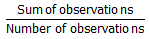(ii) Suppose a man covers a certain distance at a speed of x kmph and the same distance at y kmph. Then, the average speed during the whole journey is the harmonic mean and is equal to  2xy/(x+y) kmph.

PROPERTIES OF AVERAGE:

1. The average of the given numbers lies between the smallest and the largest number.

2. If the numbers (whose average is to be found) are at equal distance, the number in the middle of the series (after arranging them in ascending or descending order) is the average.

3. The sum of the differences of the numbers, which are less than the average, from the average is always equal to the sum of the differences of the numbers, greater than the average, from the average.

Example: 10, 12, 15, 16, 20, 35 numbers have the average as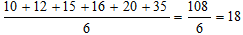Numbers 10, 12, 15 and 16 are less than the average 18. Let us find out the sum of the differences of these numbers from the average?
Solution: The differences are 18 – 10 = 8, 18 – 12 = 6, 18 – 15 = 3 18 – 16 = 2
The sum of the differences is 8 + 6 + 3 + 2 = 19
Now find out the difference of the numbers, greater than the average, from the average.
The differences are 20 – 18 = 2,  35 – 18 = 17
∴ The Sum of the differences = 2 + 17 = 19
So we find that sum of the differences of smaller numbers from average is equal to the sum of differences of larger numbers.

4. While computing average, if 0 is one of the quantities, then this should also be included in the calculation of average.

5. Unit of average is the same as that of the given quantities

Example: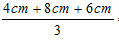=  6 cm

6. The sum of the quantities = Their average x Their number.

Example: If the average of 5 quantities is 20 (say), then the sum of these quantities is 20 x 5 = 100.

7. When a Person replaces another person in the group(age, weight, height and similar kind of Problems)

• If the average is increased: New member’s age = Age of person who left+(Increase in average*total number of People)
• If the average is decreased: New member’s age = Age of person who left - (Decrease in average*total number of People)

When someone joins the group

• Increase in average: New member’s age = Earlier average + (Increase in average*total number of People)
• Decrease in average: New member’s Age = Earlier average – (Decrease in average*total number of People)

8. If each one of the given numbers is multiplied/ divided by a number k, then the average of the given numbers will be multiplied/divided by k.

9. Average of first n natural numbers is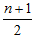10. If a person travels a distance at a speed of x km/hour and the same distance at a speed of y km/hr, then the average speed during the whole journey is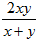11. If a person travels three equal distances at the speed of x km/hr, y km/hr, and z km/hr respectively, then the average speed during the whole journey is given by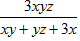km/hr.

SOLVED EXAMPLES

Example 1: A class of students consist of 36 boys and 44 girls. If the average weight of boys is 40 kg and that of girls is 35 kg, find the average weight of the whole class.
Solution: Total weight of the whole class (i.e. 36 boys + 44 girls)
= (36 x 40 + 44 x 35) kg = 2980 kg.
Average weight of the whole class = 2980/80 = 37.25 kg.

Example 2: Nine persons went to a hotel to take meals. Eight of them spent Rs 12 each and the ninth spent Rs 8 more than the average expenditure of all the nine. What was the total money spent by them.
Solution: Let the average expenditure of all the nine be Rs x
Then 12 x 8 + ( x + 8) = 9x
or 8x = 104 or  x = 13
Total money spent = 9 x = 9 X 13 = 117.

Example 3: There are 24 kids in a class and the average weight of a class is 38 kgs. If the weight of the teacher is also included then average increases by 1.5 kg. What is the weight of the teacher?
Solution: Conventional Method
Total weight of students= Average weight of students*No. of Students
= 38*24 = 912 kg
New average = 38+2 = 39.5 kg
Total number of People = 24+1 (including teacher) = 25
New total of weights= 39.5*25 = 987.5
Weight of teacher= New total of weights – Previous Total weights of Students
= 987.5-912 = 75.5 kg
Shortcut: As the question says there is an increase in the average by 1.5 kg when teacher’s weight is also considered so we will apply the formula discussed above
Teachers weight= Earlier Average+(Increase in average*Total number of people)
=  38 + (1.5*25)
=  38 + (37.5) = 75.5 kg
Hence Teacher’s weight would be 75.5 kg

See the difference in conventional and shortcut method, Due to involvement of numerous steps the conventional method becomes more time taking although it’s not too long if you are in a habit of oral number crunching but if not then it is advised to use shortcuts.

Example 4: Delhi is 805 km from Banaras. Anuj travelled from Delhi to Banaras via train at a speed of 92 km/hr whereas he took a bus to return which travelled at a speed of 54 km/hr. Find the average speed at which Anuj travelled from Delhi to Banaras and back to Delhi.
Solution: Let the speed of Train be x and speed of bus be y. By applying the Average Speed formula we get,
Average Speed = (2*x*y)/x+y
= 2*92*54 / (92+54)
= 9936/146 = 68.05 km

Example 5: A cafe has an average of 580 customers on Sundays and 250 customers on other days. Find the average number of customers per day. Consider the number of days in a month to 30.
Solution: If the number of days in a month is 30 then there would be 4 complete weeks + 2 days. 4 weeks means 4 Sundays with 580 customers thus 26 normal days with 250 customers.
Total Number of Customers = Customers on Sunday + Customers on other days
= 580*4 + 250*26 = 2320 + 6500
= 8820
Number of Days = 30
Average Number of Customers in a day=Total number of customers/Number of Days  = 8820/30 = 294 customers/day

Example 6: Average runs made by Viraj in 15 matches is 42.8. the average of his first 8 matches is 55.4. What is the average of his last 7 matches?
Solution: As we need average of Viraj’s last 7 matches run we will first find out how many runs he made in those last 7 matches by subtracting runs of his first 8 matches from total runs.
Total Runs made by Viraj in 15 matches=Average runs made*No. of matches = 42.8*15
= 642
Runs made by Viraj in first 8 marches = 55.4*8
= 443.2
Runs made by Viraj in last 7 matches = 642-443.2
= 198.8
The average number of runs made by Viraj in his last 7 matches = 198.8/7
=28.4

Example 7: The average age of 8 persons is increased by 2 years when one of them whose age is 24 years is replaced by a new person. The age of the new person is
(a) 42 years
(b) 40 years
(c) 38 years
(d) 45 years

Solution: Age of new person = 8 x 2 + 24 = 16 + 24 = 40 years.

Example 8: The average age of 30 boys in a class is 15 years. One boy, aged 20 years, left the class, but two new boys came in his place whose age differs by 5 years. If the average age of all the boys now in the class becomes 15 years, the age of the younger newcomer is:
(a) 20 years
(b) 15 years
(c) 10 years
(d) 8 years

Solutions: Age of two new boys = (15 x 31) – ( 15 x 30 – 20)
= 465 – 430= 35 years
To find the individual age of new boys:
Let their ages be x and y
X – y = 5 and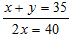or x = 20,  y = 15
Age of younger new comer is 15 years (b).

Offer running on EduRev: Apply code STAYHOME200 to get INR 200 off on our premium plan EduRev Infinity!

## Quantitative Techniques for CLAT

63 videos|22 docs|93 tests

,

,

,

,

,

,

,

,

,

,

,

,

,

,

,

,

,

,

,

,

,

;